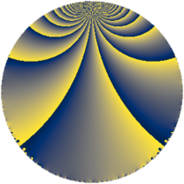# Properties

 Label 75.3.jLevel $75$ Weight $3$ Character orbit 75.j Rep. character $\chi_{75}(11,\cdot)$ Character field $\Q(\zeta_{10})$ Dimension $72$ Newform subspaces $1$ Sturm bound $30$ Trace bound $0$

# Learn more

## Defining parameters

 Level: $$N$$ $$=$$ $$75 = 3 \cdot 5^{2}$$ Weight: $$k$$ $$=$$ $$3$$ Character orbit: $$[\chi]$$ $$=$$ 75.j (of order $$10$$ and degree $$4$$) Character conductor: $$\operatorname{cond}(\chi)$$ $$=$$ $$75$$ Character field: $$\Q(\zeta_{10})$$ Newform subspaces: $$1$$ Sturm bound: $$30$$ Trace bound: $$0$$

## Dimensions

The following table gives the dimensions of various subspaces of $$M_{3}(75, [\chi])$$.

Total New Old
Modular forms 88 88 0
Cusp forms 72 72 0
Eisenstein series 16 16 0

## Trace form

 $$72q - q^{3} + 26q^{4} - 11q^{6} - 8q^{7} - 13q^{9} + O(q^{10})$$ $$72q - q^{3} + 26q^{4} - 11q^{6} - 8q^{7} - 13q^{9} - 20q^{10} + 31q^{12} - 42q^{13} + 45q^{15} - 130q^{16} + 30q^{18} - 36q^{19} - 60q^{21} - 70q^{22} - 72q^{24} + 100q^{25} - 154q^{27} - 62q^{28} + 15q^{30} + 114q^{31} - 10q^{33} + 178q^{34} + 271q^{36} - 98q^{37} - 155q^{39} - 120q^{40} - 475q^{42} - 52q^{43} + 35q^{45} + 198q^{46} - 326q^{48} + 112q^{49} + 44q^{51} + 412q^{52} + 304q^{54} + 10q^{55} + 622q^{57} + 190q^{58} + 360q^{60} - 306q^{61} + 293q^{63} + 474q^{64} + 320q^{66} + 472q^{67} + 269q^{69} - 840q^{70} + 175q^{72} + 318q^{73} - 310q^{75} + 112q^{76} + 815q^{78} - 346q^{79} - 373q^{81} - 1620q^{82} - 730q^{84} - 530q^{85} - 370q^{87} - 810q^{88} - 230q^{90} - 550q^{91} - 272q^{93} - 612q^{94} - 698q^{96} + 182q^{97} - 160q^{99} + O(q^{100})$$

## Decomposition of $$S_{3}^{\mathrm{new}}(75, [\chi])$$ into newform subspaces

Label Dim. $$A$$ Field CM Traces $q$-expansion
$$a_2$$ $$a_3$$ $$a_5$$ $$a_7$$
75.3.j.a $$72$$ $$2.044$$ None $$0$$ $$-1$$ $$0$$ $$-8$$